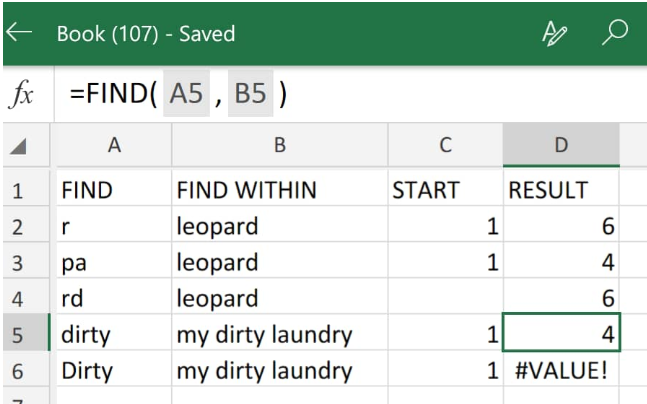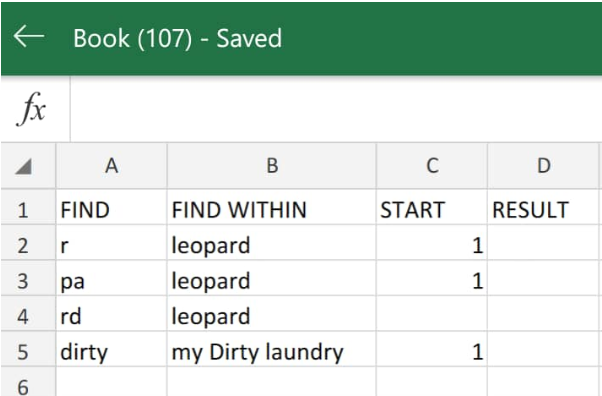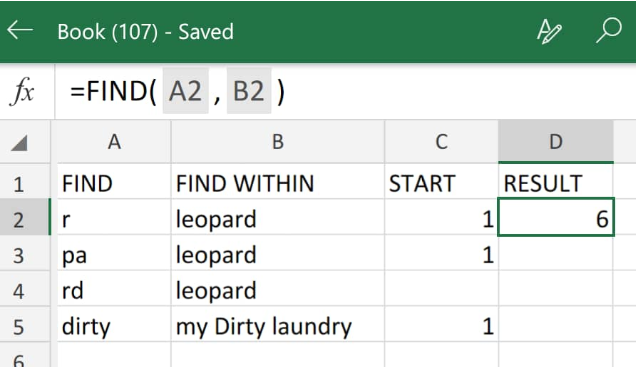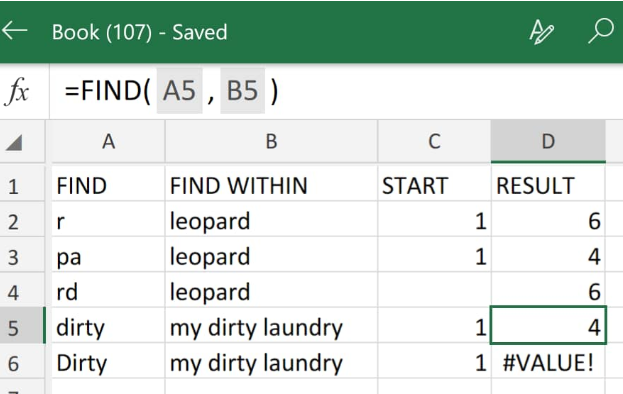Get instant live expert help with Excel or Google Sheets“My Excelchat expert helped me in less than 20 minutes, saving me what would have been 5 hours of work!”

#### Post your problem and you’ll get expert help in seconds.

Your message must be at least 40 characters
Our professional experts are available now. Your privacy is guaranteed.

# Find Function in Excel – Excelchat

The Find function in Excel determines the exact location (in number format) of a specified short text string within another longer one.

Whenever the text searched for is not found, the Excel Find formula returns the #VALUE error.Figure 1. of Find Function in Excel

## Generic Formula

`=FIND(find_text,within_text,[start_num])`

• find_text = The text we want Excel to find.
• within_text = The text string for Excel to search within.
• start_num (optional) = The starting point for Excel to begin the search (defaults is 1).

The Find or search function in Excel is case-sensitive; thus, it does not recognize wildcards such as asterisk, tilde, and question mark.

## How to Use the Find Function in Excel

We will now use the Find formula in Excel to locate a series of sub-text within larger text strings in the simple steps highlighted below;

1. We start by collecting the data available to us inside our spreadsheet;Figure 2. of Text Strings in Excel

Our goal in the illustration above is to search the text strings in column B for the values in each cell in column A.

Be sure to allocate empty cells for the search function in Excel to return the desired results (see column D above)

1. The Excel Find formula we will enter into cell D2 above is as follows;

`=FIND(A2,B2)`Figure 3. of Find Function in Excel

The find function in Excel returned “6” as the number value representing the exact location of the letter “r” inside the word “leopard”.

1. To generate similar results, modify and copy the find function in D2 down the other cells in the RESULT column;Figure 4. of Find Function in Excel

Note that the Excel Find formula returned the #VALUE error in cell D6, because of the case sensitive nature of the find function.